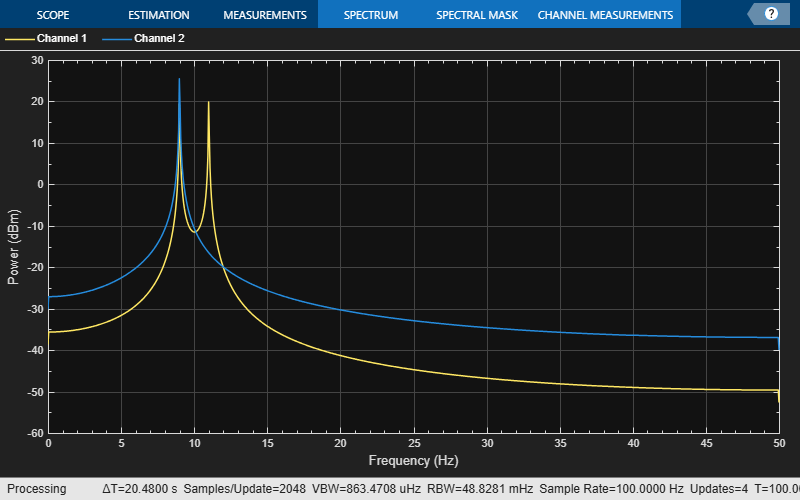Main Content

# ssbmod

Single sideband amplitude modulation

## Syntax

```y = ssbmod(x,Fc,Fs) y = ssbmod(x,Fc,Fs,ini_phase) y = ssbmod(x,fc,fs,ini_phase,'upper') ```

## Description

`y = ssbmod(x,Fc,Fs)` uses the message signal `x` to modulate a carrier signal with frequency `Fc` (Hz) using single sideband amplitude modulation in which the lower sideband is the desired sideband. The generated output `y` is a single side band signal with a suppressed carrier. The carrier signal and `x` have sample frequency `Fs` (Hz). The modulated signal has zero initial phase.

`y = ssbmod(x,Fc,Fs,ini_phase)` specifies the initial phase of the modulated signal in radians.

`y = ssbmod(x,fc,fs,ini_phase,'upper')` uses the upper sideband as the desired sideband.

## Examples

collapse all

Set the sample rate to 100 Hz. Create a time vector 100 seconds long.

```fs = 100; t = (0:1/fs:100)'; ```

Set the carrier frequency to 10 Hz. Generate a sinusoidal signal.

```fc = 10; x = sin(2*pi*t); ```

Modulate `x` using single- and double-sideband AM.

```ydouble = ammod(x,fc,fs); ysingle = ssbmod(x,fc,fs); ```

Create a spectrum analyzer object to plot the spectra of the two signals. Plot the spectrum of the double-sideband signal.

```sa = dsp.SpectrumAnalyzer('SampleRate',fs, ... 'PlotAsTwoSidedSpectrum',false, ... 'YLimits',[-60 40]); step(sa,ydouble) ```Plot the single-sideband spectrum.

```step(sa,ysingle) ```## See Also

### Topics

Introduced before R2006a

## Support

#### Bridging Wireless Communications Design and Testing with MATLAB

Download white paper